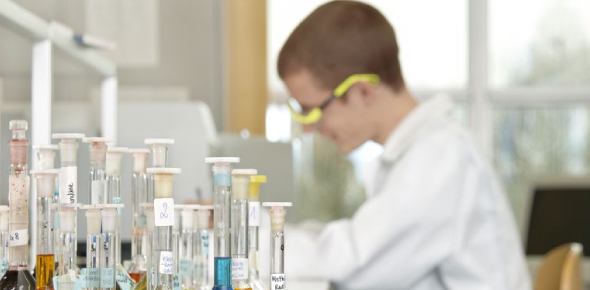# Chemistry Quiz Test

48 Questions | Total Attempts: 3345SettingsChemistry. Includes compounds, elements, formulas, ionic vs covlent, four reaction types, balancing equations (law of conservation of matter), reaction rates, acids, and bases! So I didn't know how to study for our test, so I made a quiz! sorry if i spelled something wrong, which makes you get it wrong. = ( This isn't very good, and may not have everything you need in order to study for the upcoming test, but i tried. Good luck, and if there's any suggestions, feel free to tell me! P. S. Sorry its so long, it took so long to make!

• 1.
A pure substance.
• A.

Gold

• B.

Element

• C.

Mixture

• D.

Compound

• E.

Polymer

• 2.
How many atoms are there in this chemical formula? 2Fe{sub2}(SO{sub4}){sub3}
• A.

10

• B.

25

• C.

34

• D.

52

• E.

43

• 3.
Make these into a formula. Mg+2 and HCO{sub3}-1
• A.

MgHCO{sub3}

• B.

Mg{sub5}HCO{sub2}

• C.

Mg{sub2}HCO

• D.

Mg(HCO){sub2}

• E.

Mg(HCO{sub3}){sub2}

• 4.
Check all that are true about covalent compounds [bonds]
• A.

Low melting points

• B.

Nonmetals bonding with nonmetals

• C.

Share electrons

• D.

Electrons are donated or taken based on the charge of the particle

• E.

Easily dissolve in water

• 5.
Check all that are true about ionic compounds [bonds]
• A.

Metal bond with nonmetal

• B.

Easily dissolve in water

• C.

Electrons are shared

• D.

Electrons are donated or taken based on the charge of the particle

• E.

Low melting points

• 6.
The molecule(s) on the left side of the equation are called ______________
• A.

Reactants

• B.

Products

• C.

Starters

• D.

Enders

• E.

Beginners

• 7.
The new molecule(s) on the right side of the equation are called _____________
• A.

Enders

• B.

Starters

• C.

Products

• D.

The right siders

• E.

Reactants

• 8.
Name the type of reaction: A+B yields AB
• A.

Decomposition

• B.

Double Replacement

• C.

Synthesis

• D.

Single Replacement

• 9.
Name the type of reaction: AB yields A+B
• A.

Decomposition

• B.

Double Replacement

• C.

Synthesis

• D.

Single Replacement

• 10.
Name the type of reaction: A+BC yields AC+B
• A.

Decomposition

• B.

Double Replacement

• C.

Synthesis

• D.

Single Replacement

• 11.
Name the type of reaction: AB+CD yields AD+CB
• A.

Decomposition

• B.

Double Replacement

• C.

Synthesis

• D.

Single Replacement

• 12.
Balance this equation: ___Hg +4Na yields ___HgNa{sub2}
• A.

2, 2

• B.

2,3

• C.

3,2

• D.

3,3

• 13.
According to the ____ __ ___________ __ ______, matter can not be __________ or ____________.
• A.

Law of Conservation of Matter created destroyed

• B.

S

• 14.
When a substance loses heat, but its surroundings gain heat
• A.

Exothermic

• B.

S

• 15.
When a substance gains heat, but its surroundings lose heat
• A.

Endothermic

• B.

S

• 16.
Check all that are true about acids
• A.

Bitter taste

• B.

React with metals to form H{sub2} gas

• C.

Burn/sting

• D.

Hydroxides (-OH)

• E.

Conduct electricity

• 17.
Check all that are true about bases
• A.

Feel slippery

• B.

Bitter taste

• C.

React with animal fat

• D.

Conduct electricity

• E.

Hydronium ions (H-)

• 18.
Acid+Base= ______ and water
• A.

Salt

• B.

S

• 19.
Name this type of pure carbon: Carbon tightly bound to 3 other carbon atoms in loose sheets
• A.

Diamond

• B.

Graphite

• C.

Nanostructures (Nanotubes, fullurenes)

• D.

Nanostructures (Fullurenes, nanotubes)

• 20.
Name this type of pure carbon: flexible, sturdy tubing "bucky-balls"
• A.

Diamond

• B.

Graphite

• C.

Nanostructures (Nanotubes, fullurenes)

• D.

Nanostructures (Fullurenes, nanotubes)

• 21.
A monomer is
• A.

One unit

• B.

Many units

• 22.
A polymer is
• A.

One unit

• B.

Many units

• 23.
Name a composite (you may not get the one you're supposed to get, but yours might still be right)
• A.

Wood

• B.

S

• 24.
What are Nucleic acids?
• A.

ENA

• B.

GNA

• C.

DNA

• D.

RNA

• E.

TNA

• 25.
Chains of amino acids are called _______.
• A.

Proteins

• B.

S

Related TopicsBack to top